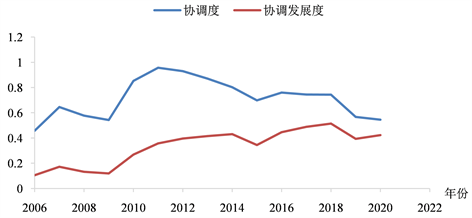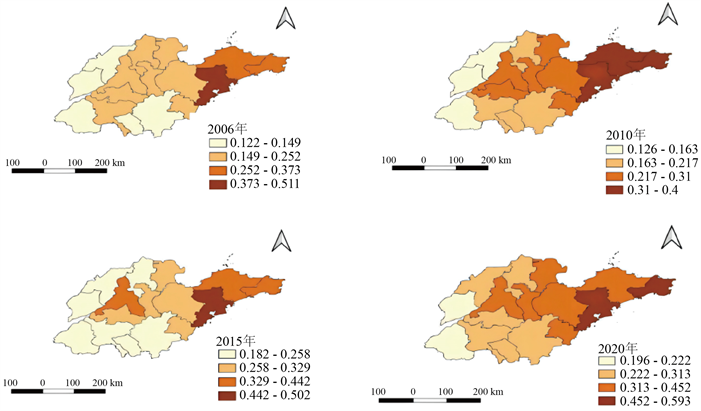#### 期刊菜单

Analysis of the Spatial and Temporal Evolution Characteristics of High-Quality Urban Development in Shandong Province—Based on Panel Data from 16 Cities in Shandong Province
DOI: 10.12677/GSER.2022.116065, PDF, HTML, XML, 下载: 29  浏览: 112

Abstract: Promoting economic shift to high-quality development is an inevitable trend in China’s future economic development. This paper analyzes the spatial and temporal evolution characteristics of 16 cities in Shandong Province from 2006 to 2020 by constructing the index system of high-quality urban development and using the entropy value method, coordination degree model and Moran index and other measurement methods. The results show that: the overall development of Shandong Province has the problem of high external dependence, simply pursuing the speed and scale of economic development, still in the rough economic development mode; the process of economic construction In the process of economic construction, resources and environmental factors are neglected; the speed of external economic development slows down and gradually shifts to other dimensions; the overall coordination and coordinated development of high-quality development are not high, and even in the last two years, it still shows a disorder; the spatial pattern of high-quality development shows the characteristics of east > central > west. Therefore, we should increase the investment in green and high-quality development, enhance the coupling and coordination among systems, and at the same time, we should make efforts to narrow the development gap among cities and municipalities by taking into account local conditions and playing the leading role of high-level cities.

1. 引言

2. 研究方法与数据来源

2.1. 评价指标体系构建

2.2. 研究方法

2.2.1. 熵值法

${Y}_{ij}=\frac{{X}_{ij}-\mathrm{min}\left({X}_{ij}\right)}{\mathrm{max}\left({X}_{ij}\right)-\mathrm{min}\left({X}_{ij}\right)}$ (1)

${Y}_{ij}=\frac{\mathrm{max}\left({X}_{ij}\right)-{X}_{ij}}{\mathrm{max}\left({X}_{ij}\right)-\mathrm{min}\left({X}_{ij}\right)}$ (2)

${E}_{j}=-\frac{1}{\mathrm{ln}\left(m\right)}\underset{i=1}{\overset{m}{\sum }}{P}_{ij}\mathrm{ln}{P}_{ij}$ (3)

${W}_{j}=\frac{1-{E}_{j}}{{\sum }_{j=1}^{n}{W}_{j}{Y}_{ij}}$ (4)

$U=\underset{j=1}{\overset{n}{\sum }}{W}_{j}{Y}_{ij}$ (5)

2.2.2. 协调发展度模型

$C={\left\{\left({U}_{1}×{U}_{2}×\cdots ×{U}_{n}\right)/{\left[\frac{{U}_{1}+{U}_{2}+\cdots +{U}_{p}}{P}\right]}^{P}\right\}}^{K}$ (6)

$D=\sqrt{C×T}$ (7)Table 1. Standard for classification of coupling coordination degree

2.2.3. 莫兰指数

$I=\frac{{\sum }_{i=1}^{n}{\sum }_{j-1}^{n}{W}_{ij}\left({X}_{i}-\stackrel{¯}{X}\right)\left({X}_{j}-\stackrel{¯}{X}\right)}{{S}^{2}{\sum }_{i=1}^{n}{\sum }_{j-1}^{n}{W}_{ij}}$ (8)

${I}_{i}=\frac{{X}_{j}-\stackrel{¯}{X}}{{S}^{2}}\underset{i=1}{\overset{n}{\sum }}\left[{W}_{ij}\left({X}_{i}-\stackrel{¯}{X}\right)\right]$ (9)

Moran’s I的取值范围为[−1, 1]，指数绝对值越大说明空间相关性越高。指数值通过标准Z统计量检验其是否显著。

2.3. 数据来源

3. 山东省城市高质量发展时序演化特征

3.1. 指标权重分析Table 2. High quality development indicators weighting

3.2. 综合发展指数演变趋势Table 3. Comprehensive index score table for each year

2006~2018年高质量发展各子系统协调度发展参差不齐，因为子系统间协调度容易受到各方面因素的影响，导致无法准确衡量地区发展的真实状况。但在引入发展度后，协调发展指标在2006~2020年数据虽有一定程度的波动但整体处于持续上升状态。同时，由表4可知，山东省高质量协调发展度由2006年的0.1055上升到2020年的0.4240，年均增长率保持在9.71%。这表明山东省协调发展整体发展态势较好，但发展缓慢。从发展协调度类型来看，由图1所示，山东省目前协调发展度整体处于低水平协调状态，在2020年也依然处在濒临失调状态。这表明全省的发展更侧重于数量的发展，而忽视了质量的提升，城市高质量发展处于初级阶段，协调发展度亟待进一步增强。Figure 1. Contrast scatter plot between degree of coordination and degree of coordination developmentTable 4. Coupling of subsystems

4. 空间分异特征Table 5. Global autocorrelation analysis resultsFigure 2. High quality development space level

5. 结论与讨论

5.1. 结论

5.2. 讨论

  Johnston, D., Lowe, R. and Bell, M. (2005) An Exploration of the Technical Feasibility of Achieving CO2 Emission Reductions in Excess of 60% within the UK Housing Stock by the Year 2050. Energy Policy, 33, 1643-1659. https://doi.org/10.1016/j.enpol.2004.02.003  Carfi, D. and Schiliròc, D. (2012) A Competitive Model for the Green Economy. Economic Modelling, 29, 1215-1219. https://doi.org/10.1016/j.econmod.2012.04.005  Barbier, E.B. (2010) A Global Green New Deal: Rethinking the Economic Recovery. Cambridge University Press, London. https://doi.org/10.1017/CBO9780511844607  Sippel, M. and Michaelowa, A. (2013) Financing a Green Urban Economy: The Potential of the Clean Development Mechanism (CDM). In: Simpson, R., Zimmermann, M., Eds., The Economy of Green Cities. Local Sustainability, Vol. 3, Springer, Dordrecht, 363-368. https://doi.org/10.1007/978-94-007-1969-9_31  郭付友, 吕晓, 于伟, 等. 山东省绿色发展水平绩效评价与驱动机制——基于17地市面板数据[J]. 地理科学, 2020, 40(2): 200-210. https://doi.org/10.13249/j.cnki.sgs.2020.02.005  金昌东, 张宝雷, 康洁铭. 经济高质量发展水平及其耦合协调性研究——基于山东省17地市面板数据[J]. 生态经济, 2021, 37(7): 65-72.  黄跃, 李琳. 中国城市群绿色发展水平综合测度与时空演化[J]. 地理研究, 2017, 36(7): 1309-1322.  穆学英, 刘凯, 任建兰. 中国绿色生产效率区域差异及空间格局演变[J]. 地理科学进展, 2017, 36(8): 1006-1014.  李磊, 张贵祥. 京津冀城市群内城市发展质量[J]. 经济地理, 2015, 35(5): 61-64+8. https://doi.org/10.15957/j.cnki.jjdl.2015.05.009  郭付友, 佟连军, 魏强, 张慧敏, 仇方道, 佟伟铭. 吉林省松花江流域产业系统环境适应性时空分异与影响因素[J]. 地理学报, 2016, 71(3): 459-470.  王竹君, 任保平. 基于高质量发展的地区经济效率测度及其环境因素分析[J]. 河北经贸大学学报, 2018, 39(4): 8-16. https://doi.org/10.14178/j.cnki.issn1007-2101.2018.04.002  陈明星, 陆大道, 张华. 中国城市化水平的综合测度及其动力因子分析[J]. 地理学报, 2009, 64(4): 387-398.  袁文华, 李建春, 刘呈庆, 吴美玉. 城市绿色发展评价体系及空间效应研究——基于山东省17地市时空面板数据的实证分析[J]. 华东经济管理, 2017, 31(5): 19-27.  王国力, 房娟. 山东省经济高质量发展水平测度及驱动机制分析[J]. 开发研究, 2021(6): 34-42. https://doi.org/10.13483/j.cnki.kfyj.2021.06.005  孙久文, 蒋治, 胡俊彦. 新时代中国城市高质量发展的时空演进格局与驱动因素[J]. 地理研究, 2022, 41(7): 1864-1882.  郭伟, 闫绪娴, 范玲. 中国省域经济高质量发展评估与驱动因素研究[J]. 东岳论丛, 2022, 43(7): 155-164. https://doi.org/10.15981/j.cnki.dongyueluncong.2022.07.019  孙培蕾, 郭泽华. 中国省域经济高质量发展时空格局及驱动因素[J]. 兰州财经大学学报, 2021, 37(4): 54-66.  党兴华, 赵璟, 张迎旭. 城市群协调发展评价理论与方法研究[J]. 当代经济科学, 2007(6): 110-115+126.  焦琳惠, 谢小飞, 吕剑平. 区域高质量发展的时空演变及驱动因素分析——以甘青宁地区为例[J]. 国土与自然资源研究, 2022(1): 26-31. https://doi.org/10.16202/j.cnki.tnrs.2022.01.008  程永生, 张德元, 赵梦婵. 黄河流域生态保护和高质量发展的时空演变与驱动因素[J]. 经济体制改革, 2021(5): 61-69.  汪侠, 徐晓红. 长江经济带经济高质量发展的时空演变与区域差距[J]. 经济地理, 2020, 40(3): 5-15. https://doi.org/10.15957/j.cnki.jjdl.2020.03.002  任保平, 李禹墨. 新时代我国高质量发展评判体系的构建及其转型路径[J]. 陕西师范大学学报(哲学社会科学版), 2018, 47(3): 105-113. https://doi.org/10.15983/j.cnki.sxss.2018.0421  黄明均. 长江经济带经济发展质量的时空演变特征与驱动因素分析[D]: [硕士学位论文]. 杭州: 浙江工业大学, 2019.  张震, 刘雪梦. 新时代我国15个副省级城市经济高质量发展评价体系构建与测度[J]. 经济问题探索, 2019(6): 20-31+70.  师博, 张冰瑶. 全国地级以上城市经济高质量发展测度与分析[J]. 社会科学研究, 2019(3): 19-27.